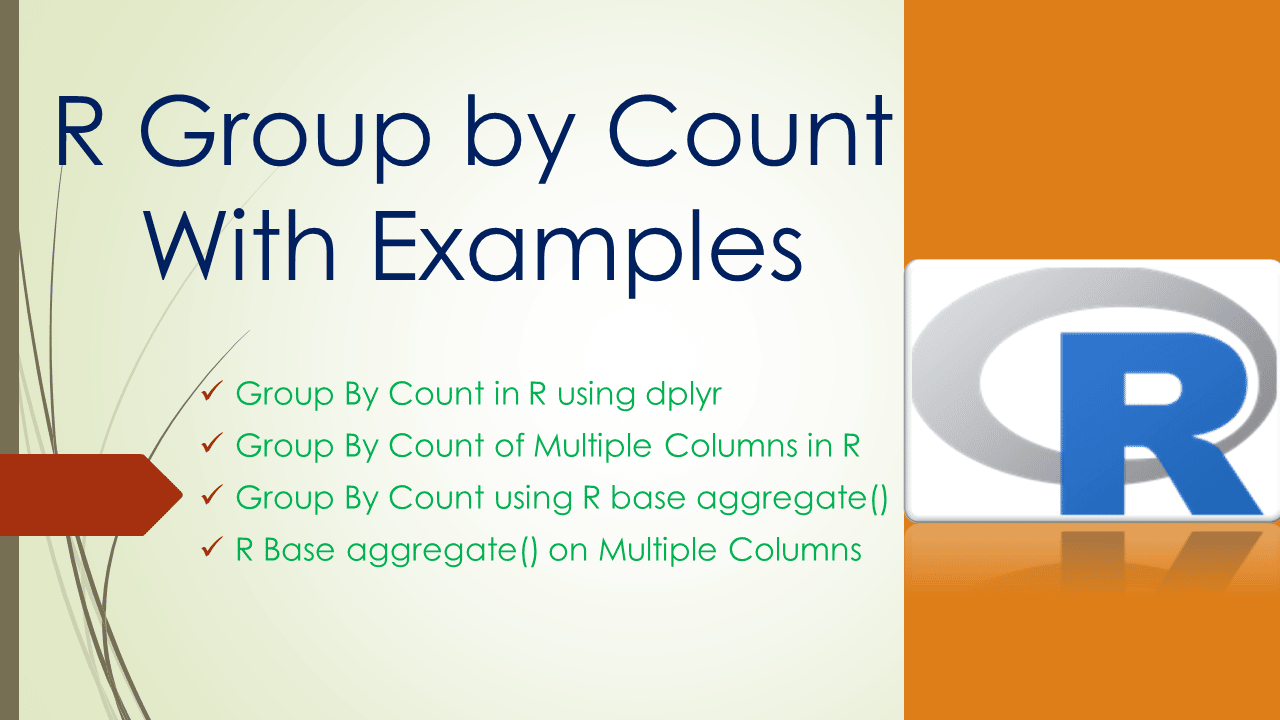# R Group by Count With Examples

Ho to do group by count in R? By using aggregate() from R base or `group_by()` function along with the summarise() from the dplyr package you can do the group by on dataframe rows based on a column and get the count for each group.

Since using the group_by() function from the dplyr package is an efficient approach, I will cover this first and then use the aggregate() function from the R base.

## 1. Quick Examples

Following are quick examples of how to perform group by count.

``````
library(dplyr)

# Group by count using dplyr
agg_tbl <- df %>% group_by(department) %>%
summarise(total_count=n(),
.groups = 'drop')

# Convert tibble to df
df2 <- agg_tbl %>% as.data.frame()

# Group by count of multiple columns
df2 <- df %>% group_by(department,state) %>%
summarise(total_count=n(),.groups = 'drop') %>%
as.data.frame()

# Group by count using R Base aggregate()
agg_df <- aggregate(df\$state, by=list(df\$department), FUN=length)

# R Base aggregate() on multiple columns
agg_df <- aggregate(df\$state, by=list(df\$department,df\$state), FUN=length)
``````
``````
# Read CSV file into DataFrame
df
``````

Yields below output.

## 2. Group By Count in R using dplyr

You can use `group_by()` function along with the `summarise()` from dplyr package to find the group by count in R DataFrame, `group_by()` returns the grouped_df ( A grouped Data Frame) and use summarise() on grouped df to get the group by count.

To use these functions first, you have to install dplyr first using install.packages(‘dplyr’) and load it using `library(dplyr)`. I will use dplyr infix operator `%>%` across all our examples as the result of group_by() function goes as input to summarise() function.

``````
library(dplyr)

# Group by count using dplyr
agg_tbl <- df %>% group_by(department) %>%
summarise(total_count=n(),
.groups = 'drop')
agg_tbl

# Convert tibble to df
df2 <- agg_tbl %>% as.data.frame()
df2
``````

Yields below output. The following example does the group by on department column and get the count for each department.

Note that `group_by()` and `summarise()` function returns tibble, if you want DataFrame you should convert tibble to dataframe by using as.data.frame().

## 3. Group By Count of Multiple Columns in R

The following example does the group by of department and state columns and get the count for each department & state combination.

``````
# Group by count of multiple columns
df2 <- df %>% group_by(department,state) %>%
summarise(total_count=n(),.groups = 'drop') %>%
as.data.frame()
df2
``````

Yields below output.

## 4. Group By Count using R base aggregate()

R base provides an aggregate() function to perform the grouping on the dataframe, let’s use this to perform a groupby on the department column and get the count for each department.

``````
# Group by count using R Base aggregate()
agg_df <- aggregate(df\$state, by=list(df\$department), FUN=length)
agg_df
``````

Yields below output.

## 5. R Base aggregate() on Multiple Columns

The following example uses the aggregate() function to group rows based on department and state columns and uses the length function to get the count for each department & state combination.

``````
# R Base aggregate() on multiple columns
agg_df <- aggregate(df\$state, by=list(df\$department,df\$state), FUN=length)
agg_df
``````

Yields below output.

## Conclusion

In this article, I have explained how to do group by count in R by using group_by() function from the dplyr package and aggregate function from the R base. Between these two, dplyr functions perform efficiently when you are dealing with larger datasets.

## References

### Naveen (NNK)

Naveen (NNK) is a Data Engineer with 20+ years of experience in transforming data into actionable insights. Over the years, He has honed his expertise in designing, implementing, and maintaining data pipelines with frameworks like Apache Spark, PySpark, Pandas, R, Hive and Machine Learning. Naveen journey in the field of data engineering has been a continuous learning, innovation, and a strong commitment to data integrity. In this blog, he shares his experiences with the data as he come across. Follow Naveen @ @ LinkedIn